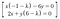# Finding eigenvectors and eigenvalues. Step-by-step.

I hope every data scientists knows how eigenvectors and eigenvalues are important in ML/DS area. But this post is rather for people who start studying data science from scratch and I would like to put my contribution just to help someone who seeks a simple answer. I won’t dwell on theory because I there are a lot of materials about the topic we will discuss in web, just want to focus on example.

The equation of a linear transformation:

Let’s look at the example a bit closer. Supposed, we have a matrix A:

That means that there is eigenvalues and eigenvectors that satisfy such equation:

If we apply matrix multiplication and draw up system of equations it will result in the following expression:

Apparently, we can express matrix as a system of equatioins for reducing complexity:Source equation

Of course, you can omit these steps and write matrix immediately but that was important to know why we have lambda in diagonal. Let’s write a matrix, but be careful with x and y coefficients. Vector v cannot be zero. That means, x and y cannot have a trivial solution when x = 0 and y = 0. In this case the equations are lineary dependent (we will see it later) and a matrix determinant is equal to zero.

That is called characteristic equation of the matrix A. Let’s find the determinant and hence find the eignent values from equation:

Substitute these values to our equation (see Source equation above)

If there is no linear dependency (in our case x=y=0), then there in a mistakes in calculations. If we place any x for y (or vise versa) it will result in any infinite number of eigenvectors. That is why we can choose any y. Example suggests to use y=-1 just for simplicity.

Do the same for another lambda (y=-2)

We have found eigenvectors and eigenvalues.

## More from dmytro.pyvovarenko

LinkedIn: https://www.linkedin.com/in/dmytro-pyvovarenko-558285125/